# Question about RDF command and how it is done

Dear Coordinator

I hope this email finds you well

The question that I have is related to the simulation of a NaCl like structure system, two infused FCC that form a Simple Cubic, that are interacting with each other with Lj potential. When I calculate the RDF of the system, at first before applying the npt as follows, my RDF for a-a and a-b are fine as you can see in the first two graph. I should say that by fine I mean the ration between first and second peaks is equal to the ratio that I expected.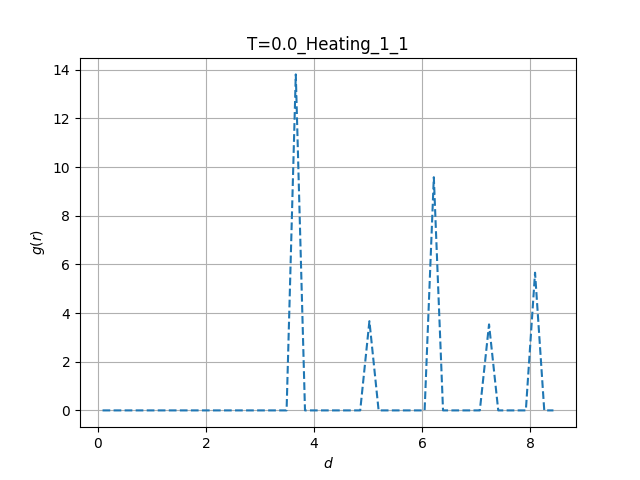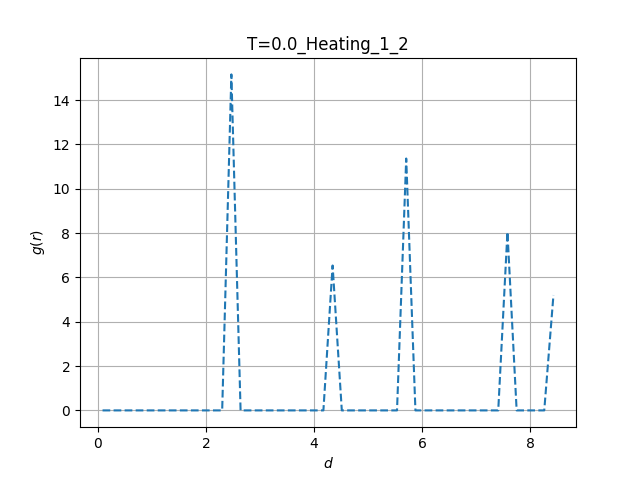However, after I apply the npt to the system, the rdf and the ratio goes to some value different to what I expect. Later, I found out that the position of the first peak is as the same as the value for the \sigma for the potential between two type. I should say for the result in this email the Lj Parameter \sigma for a-b interaction is the half of the one for a-a and a-b.

the problem that I have is that it doesnt make sense since I use the Periodic boundary condition and I cannot understand how this could happen spatially.

Hamid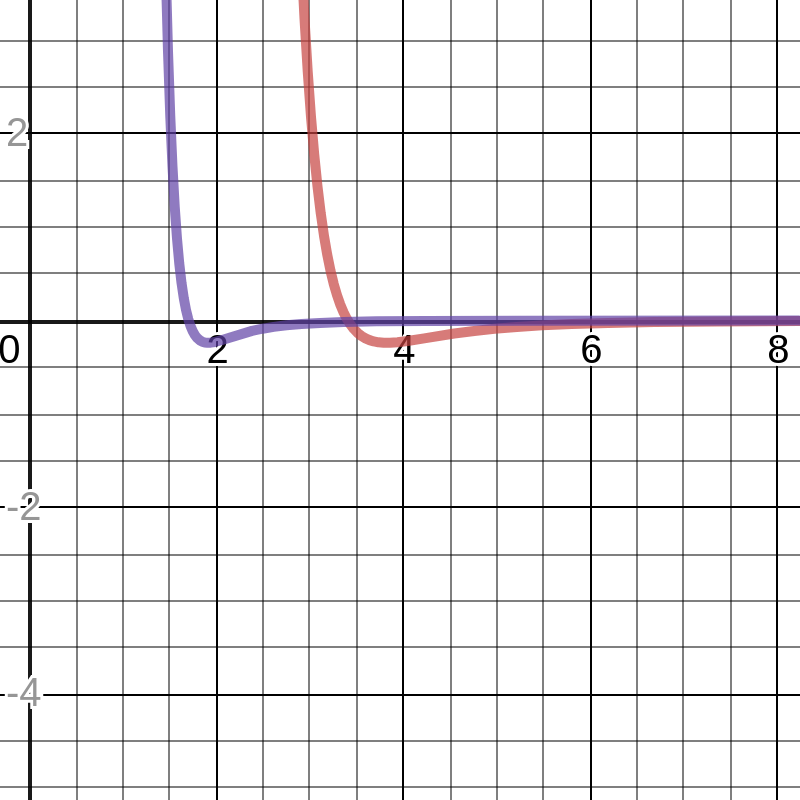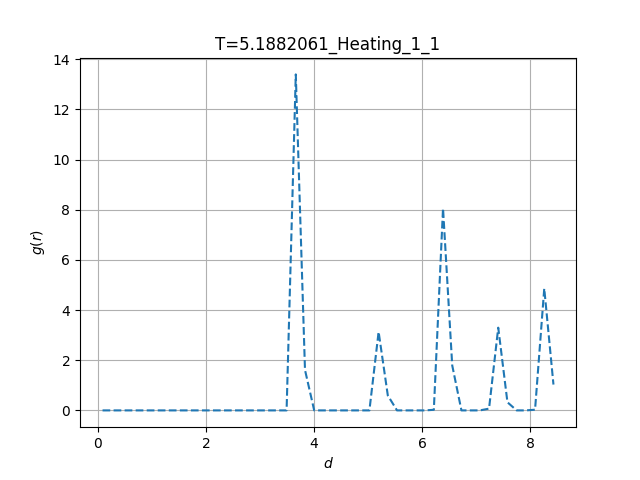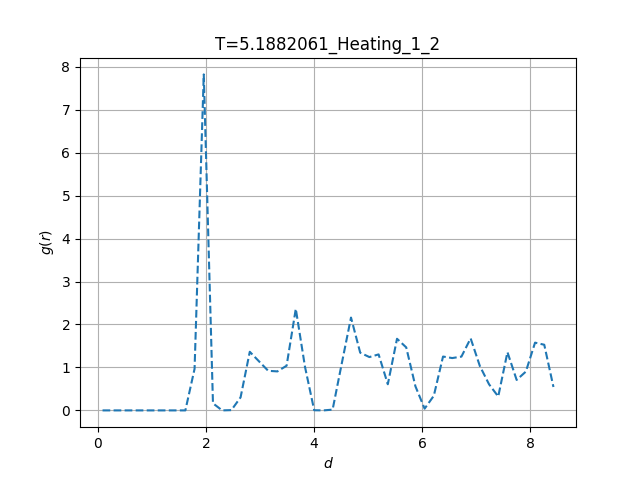fix ENS all npt temp 0.01 452.40